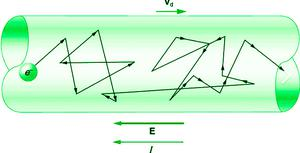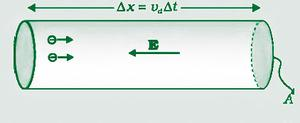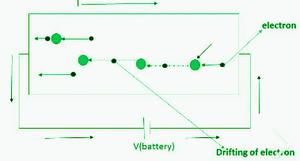GFG App
Open AppBrowser
Continue

A vector measurement of the rate and direction of motion is defined as velocity. Simply put, velocity refers to how fast something moves in one direction. Velocity may be used to calculate the speed of a car heading north on a major highway and the speed of a rocket launching into space. In this topic, let’s learn about Drift velocity.

### Drift velocity

The name is drift velocity because the electron drifts in the opposite direction of the electric field at low velocity and at high thermal velocities between two collisions. The drift velocity refers to the average velocity obtained by a particle, such as an electron, as a result of an electric field’s action. Because the movement or motion of the particle is believed to be in a plane, the axial drift velocity can be used to describe the motion.

Drift velocity is defined as “The Average velocity with which the free electrons get drifted towards the positive end of the conductor under the influence of an external electric field.”The random motion of free electrons moving around in the conductor can also be used to understand drift velocity. The electrons continue to move randomly as a result of this field, but their random motion will shift them towards a higher potential. This indicates that the electrons are drifting towards the conductor’s higher potential end. As a result, each electron will have a net velocity toward the conductor’s end. The Drift current is the current generated by the motion of electrons inside a conductor.

Because of collisions with other particles in the conductor, charged particles moving around in a conductor do not move in a straight line. As a result, the average particle speed in the conductor is considered. The drift velocity is the word for this.

• The drift velocity of electrons is in the order of 10-4 ms-1.
• The external electric field applied to the conductor set up is if V is the potential difference applied across the ends of the conductor of length l.

E = Potential difference / length = V / l

#### Derivation

• Each free electron in the conductor experience a force,

F = -eE

• The acceleration of each electron is

a = F / m

F = -eE

∴ a = -eE / m (where m = mass of electron)

• At any instant of time, the velocity acquired by electron having thermal velocity u1 will be,

v1 = u1 + aτ1 (using v = u + at)

Where, τ1 = time taken by the electron to collide with positive ion, v1 = initial velocity.

Similarly, velocity acquired by electron, v2 = u2 + aτ2 , v2 = u2 + aτ2 , . . . . . . , vn = un + aτn

• The average velocity of all the free electrons in the conductor under the effect of the external electric field is the drift velocity vd of the free electrons.

vd = ( v1 + v2 + . . . . + vn ) / n

= ((u1 + aτ1) + (u2 + aτ2) + . . . . + (un + aτn)) / n

= ((u1 + u2 + . . . +un)/n) + a((τ1 + τ2 + . . . . . + τn) /n)   …(equation 1)

when Electric field is not applied on conductor then current result is not in metal.

∴ (( u1 + u2 + . . . + un )/n) =0  …(equation 2)

put equation 2 in equation 1. Therefore,

= 0 + aτ     …(where τ = ((τ1 + τ2 + . . . . . + τn) /n))

Average relaxation time refers to the length each electron takes to recover from its most recent interaction with a conductor atom.

• Value is the order of 10-14 seconds.

∴ vd = aτ

we know, a = -eE / m

∴ vd = -eEτ / m

Average drift speed, vd = eEτ / m  …(equation 3)

where, e = charge of electron, E = Electric field, τ = Relaxation time, m = mass of electron. Here, e and m are constant.

(Negative sign shows that vd is opposite to the direction of E.)• Due to the frequent collisions that electrons have, their drift velocity is small.
• If the cross-section is constant, i μ J i.e for a given cross-section area, the greater the current density, the larger will be current.
• In the presence of an exceptionally large number of free electrons in a conductor, a tiny amount of drift velocity produces a large amount of electric current.
• The electric bulb glows immediately when the switch is turned on because current transmission is practically as fast as light and involves electromagnetic processes.
• In the presence of the electric field, the path of electrons between successive collision are curved and In absence of an electric field, the path of electrons between successive collision is a straight line.
• Free electron density in metal is

n = (NA × d) / A

(where, NA = Avogadro number , x = number of free electrons per atom, d= density of metal , A = Atomic weight)

Example of Drift velocity

Drift Velocity Example If any three of the four quantities are known, then the missing amount can be easily calculated, as stated in the formula above for the drift velocity. As a result, the electron drift velocity in a piece of metal with a current of 0.1 A will be roughly 1 × 10-5 m/s.

### Relaxation time (τ)

The time interval between two successive collisions of electrons with the positive ions in the metallic lattice is defined as relaxation time.

τ = mean free path / r.m.s. velocity of electrons

τ = λ / vrms

τ is inversely proportional to vrms (∴ when vrms increases consequently then τ decreases.)

### Mobility (μ)

Electron mobility is defined as “drift velocity per unit electric field.

i.e. μ = vd / E

Its unit is m2 / volt-sec

Therefore, ohm’s law can be explained in terms of drift velocity as,

vd = μE

These quantities SI unit is m/s. It is also measured in m2/(V.s)

The magnitude of charge drift velocity per unit electric field applied is specified as the mobility of the charge carrier responsible for current.

i.e   μ = drift velocity / Electric field

μ = vd / E

μ = (eEτ / m) / E …(from equation 3)

mobility of electron, μ = eτ / m

SI unit of mobility μ is m2S-1V-1 or ms-1N-1C

### Relation between Current and Drift velocity

Current (i) is a rate of flow of charge.

Current (i) = Q / t  ⇢ (equation 4)

Let, length of wire = l, Area of cross-section = A, Number of electron volume = n, Drift velocity = vd

In simple, vd = l / t

∴ t = l / vd  ⇢ (equation a)

Volume of conductor = A × l

If n is the number density of electrons, i.e the number of free electrons per unit volume of the conductor, then the total number of a free electron in the conductor is,

Electron = A × l × n

Then total charge on all the free electrons in the conductor,

Charge(Q) = Number of electron × charge of each electron

∴ Q = A × l × n × e ⇢ (equation b)

As a result of this field, the free electrons in the conductor will begin to drift to the left, as indicated in the diagram below.Put equation a and equation b in equation 1. Therefore, equation 1 becomes,

i = (A × l × n × e) / (l/vd)

∴ i = A × l × n × e × vd / l

i = A × n × e × vd  ⇢ (equation 5)

The average velocity gained by the free electrons of the conductor, which the electrons get drift under the influence of electric field is given as,

vd = i / A × n × e ⇢ (equation 6)

Putting the value of Average drift velocity vd = eEτ / m in equation 5,

i = A × n × e2 × τ × E / m

### Relation between Current Density and Drift velocity

The current density is defined as ” The whole current supplying throughout a cross-sectional conductor unit within a unit of time.

i = A × n × e × vd  ⇢ (equation m)

∴ J = i /A ⇢ (equation 7)

Put equation m in equation 7,

J = A × n × e × vd / A

∴ J = nevd

Where, J is density of current, Area of cross-section = A, Number of electron volume = n, Drift velocity = vd , e = charge.

Therefore, the electron velocity and its current density are equivalent to each other.

• When an electric field is applied across a conductor, the electrons shift towards the high potential end of the wire.
• The current flowing in a conductor is directly proportional to the drift velocity of electrons.
• Unless an electric field is provided to the conductor, the electrons inside it move at random velocities and in random directions.
• Drift velocity is directly proportional to current and inversely proportional to r2.

### Sample problems

Question 1: What is meant by drift velocity?

Drift velocity is defined as “The Average velocity with which the free electrons get drifted towards the positive end of the conductor under the influence of an external electric field.”

Question 2: The drift velocity of a free electron in a conductor is v when a current I is flowing in it. If both the radius and current are doubled, then the drift velocity will be?

Given :  vd1 = v, i1 = i, i2 = 2i, r1 = r, r2 = 2r

To Find: vd2

Solution:

We know, i = A × n × e × vd

∴ vd = i / A × n × e

∴ vd = i / (πr2) × n × e

Drift velocity is directly proportional to current and inversely proportional to r2.

∴ vd1 / vd2  = (i1 / i2) × (r1 / r2)2

∴ vd1 / vd2 = (i / 2i) × (r / 2r)2

∴ v / vd2 = 1/2 × 4

∴ v / vd2 = 2

∴ vd2 = v / 2

∴ drift velocity is v / 2.

Question 3: A conductor wire having 1029 free electron/m3 carries a current of 20 A. If the cross-section of the wire is 1 mm2, then the drift velocity of the electron will be? (e = 1.6 x 10-19 C)

Given: n = 1029 m-3, i = 20A, A = 1 mm2 = 10-6 m2, e = 1.6 × 10-19 C

To Find: vd

Solution:

We know, i = A × n × e × vd

∴ vd = i / A × n × e

∴ vd = 20 / 10-6 × 1029 × 1.6 × 10-19

∴ vd = 1.25 × 10-3 m/s

Question 4: A copper wire has a cross-sectional area of 7.85 x 10-7 m2. The number density of copper is 8.5 x 1028 m-3. Calculate the mean drift velocity of the electrons through the wire when the current is 1.4 A.

Given : A = 7.85 × 10-7 m2 , i= 1.4 A, n = 8.5 × 1028 m-3 , e = 1.6 × 10-19

To find: mean drift velocity,

we know, I = nAvde

∴ mean drift velocity = vd = I/(nAe)

∴ vd = 1.4 / 8.5 × 1028 × 7.85 × 10-7 × 1.6 × 10-19

∴ vd = 1.31 × 10-4 m/s

Question 5: Explain the Relaxation time.

“The time interval between two successive collisions of electrons with the positive ions in the metallic lattice is defined as relaxation time.”

τ = mean free path / r.m.s. velocity of electrons

τ = λ / vrms

Question 6: Does Drift velocity depends on Diameter?

Drift velocity does not depend on the cross-sectional area or the diameter of any conductor. For the same potential difference here, with V across the conductor, an increase in the cross-sectional area decreases the resistance R=(ρ.l) / (A), And so, increasing the current i=V/R.

Question 7: When a potential difference V is applied across a conductor at a temperature T, the drift velocity of electrons is proportional to?

We know that,

Drift velocity vd = eEτ / m

∴ vd =  (e × (V/l) × τ) / m  …(E = V/l)

so for a particular conductor of a particular length the drift velocity will directly depend upon voltage. Hence, vd α V.

Question 8: The resistivity of silver (ρ) = 1.54 × 10-8 Ωm, Electric field along the wire(E) = 1 volt/cm or 100 volts/m, The carrier concentration of electron (n) = 5.8 × 1028 m-3. Find mobility of the electron and drift velocity.

Solution:

μ = σ /ne

∴ μ = 1 / ρne  …(σ = 1/ ρ)

∴ μ = 1/ 1.54 × 10-8 × 5.8 × 1028 × 1.6 × 10-19

∴ μ = 6.9973 × 10-3 m2/v/s

We know, μ = vd / E

∴ vd = μE

∴ vd = 6.9973 × 10-3 × 100

∴ vd = 0.69973 m/s

Drift velocity = 0.69973 m/s

My Personal Notes arrow_drop_up